Courses

# NCERT Solutions(Part- 2)- Understanding Quadrilaterals Class 8 Notes | EduRev

## Class 8 : NCERT Solutions(Part- 2)- Understanding Quadrilaterals Class 8 Notes | EduRev

The document NCERT Solutions(Part- 2)- Understanding Quadrilaterals Class 8 Notes | EduRev is a part of the Class 8 Course Mathematics (Maths) Class 8.
All you need of Class 8 at this link: Class 8

Question 1: Take a regular hexagon as shown in the figures:
1. What is the sum of the measures of its exterior angles x, y, z, p, q, r?
2. Is x = y = z = p = q = r?  Why?
3. What is the measure of each?

(i) exterior angle (ii) interior angle
4. Repeat this activity for the cases of

(i) A regular octagon
(ii) a regular 20-gon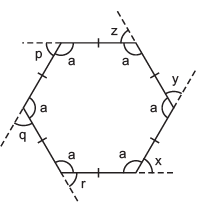Solution: 1. ∠x + ∠y + ∠z + ∠p + ∠q + ∠r = 360°  [∵ Sum of exterior angles of a polygon = 360°]
2. Since, all the sides of the polygon are equal.
∴ It is a regular hexagon.
So, its interior angles are equal.
∴  x = (180° – a)      y = (180° – a)
z = (180° – a)         p = (180° – a)
q = (180° – a)        r = (180° – a)

∴   x = y = z = p = q = r
3. (i) ∵ x + y + z + p + q + r = 360°        [∵ sum of exterior angles = 360°]
and all these angles are equal
∴ Measure of each exterior angle = 360°/6 = 60°
(ii) ∵ Exterior angle = 60°
∴  180° – 60° = Interior angle
or   120° = Interior angle
or  Measure of interior angle = 120°
4. (i) In a regular octagon, number of sides (n) = 8
∴ Each exterior angle = 360°/8 = 45°
∴  Each interior angle = 180° – 45° = 135°

(ii) For a regular 20-gon, the number of sides (n) = 20
∴ Each exterior angle = 36°/2 = 18°

Thus, each interior angle = 180° – 18° = 162°

Example 2. Find the number of sides of a regular polygon whose each exterior angle has a measure of 40°.
Solution: Since, the given polygon is a regular polygon.

∴ Its each exterior angles is equal.
∵ Sum of all the exterior angles = 360°
∴ Number of exterior angles = 360°/40° = 9
⇒ Numbers of sides = 9

Thus, its is a nonagon.

EXERCISE 3.2
Question 1. Find x in the following figures.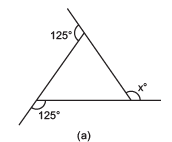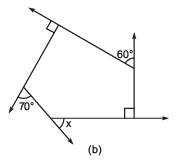Solution: (a) Sum of all the exterior angles of a polygon = 360°

∴  125° + 125° + x = 360°
or  250° + x = 360°
or   x = 360° – 250° =110°
(b) ∵ x + 90° + 60° + 90° + 70° = 360°
or  x + 310° = 360°
or  x = 360° – 310° = 50°

Question 2. Find the measure of each exterior angle of a regular polygon of
(i) 9 sides
(ii) 15 sides
Solution: (i) Number of sides (n) = 9
∴ Number of exterior angles = 9
Since, sum of all the exterior angles = 360°
∵ The given polygon is a regular polygon.
∴  All the exterior angles are equal.

∴ Measure of an exterior angle = 360°/9° = 40°
(ii) Number sides of regular polygon = 15
∴  Number of equal exterior angles = 15 The sum of all the exterior angles = 360°
∴  The measure of each exterior angle = 360°/15 = 24°

Question 3. How many sides does a regular polygon have if the measure of an exterior angle is 24°?
Solution: For a regular polygon, measure of each angle is equal.

∴ Sum of all the exterior angles = 360° Measure of an exterior angle = 24°
∴ Number of angles = 60°/24° = 15
Thus, there are 15 sides of the polygon.

Question 4. How many sides does a regular polygon have if each of its interior angles is 165°?
Solution: The given polygon is regular polygon.
Each interior angle = 165°
∴ Each exterior angle = 180° – 165° = 15°

∴ Number of sides = 360°/15° = 24°
Thus, there are 24 sides of the polygon.

Question 5. (a) Is it possible to have a regular polygon with measure of each exterior angle is 22°?
(b) Can it be an interior angle of a regular polygon? Why?
Solution: (a) Each exterior angle = 22°

∴  Number of sides = 360°/22° = 180/11
If it is a regular polygon, then its number of sides must be a whole number.
Here, 180/11 is not a whole number.

∴ 22° cannot be an exterior angle of a regular polygon.
(b) If 22° is an interior angle, then 180° – 22°, i.e. 158° is exterior angle.
∴ Number of sides = 360°/158° = 180°/79°

Which is not a whole number.
Thus, 22° cannot be an interior angle of a regular polygon.

Question 6. (a) What is the minimum interior angle possible for a regular polygon? Why?
(b) What is the maximum exterior angle possible for a regular polygon?
Solution: (a) The minimum number of sides of a polygon = 3
The regular polygon of 3-sides is an equilateral.
∵ Each interior angle of an equilateral triangle = 60°

Hence, the minimum possible interior angle of a polynomial = 60°
(b) ∵ The sum of an exterior angle and its corresponding interior angle is 180°.
And minimum interior angle of a regular polygon = 60°
∴ The maximum exterior angle of a regular polygon = 180° – 60° = 120°

Question 7. In the figure, PQRS is a parallelogram. Find the values of x, y and z.
Solution: Using the property, ‘Opposite angles of a parallelogram are equal’, we have:
z = 110°

Since, sum of all the angles of parallelogram = 360°
∴   2z + 2x = 360°
or   2(110°) + 2x = 360°
or   220° + 2x = 360° or 2x = 360° – 220° = 140°
or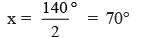Now,   y + z = 180°       [Adjacent angles of a || gm are Supplementary]
or   y + 110° = 180°
∴  y = 180° – 110° = 70°
Thus,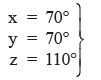Question 8. In a parallelogram JKLM, if m∠K = 80°, find all other angles.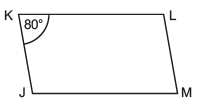Solution: Using the property, “Opposite angles of a parallelogram are equal”, we have:
m∠K= m∠M
or m∠M = 80°
Again, m∠J + m∠L + m∠K + m∠M = 360° [Sum of all angles of a quadrilateral is 360°]
or  m∠J + m∠L + 80° + 80° = 360°
or  m∠J + m∠L = 360° – 80° – 80° = 200°
But  m∠J = m∠L   [Opposite angles of parallelogram]
∴  m∠J= m∠L = 200°/2 = 100°
Thus, m∠J = 100°, m∠L = 100° and m∠M = 80°.

Question 9: After showing m∠R = m∠N = 70°, can you find m∠I and m∠G by any other method?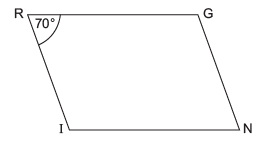Solution: Yes, without using the property of parallelogram, we can also solve to find m∠I and m∠G as given below:

∵ m∠R= m∠N = 70°
and RG || IN, the transversal RI intersecting them.
∴ m∠R + m∠I = 180° [Sum of interior opposite angles is 180°]
or   70° + m∠I = 180°
m∠I = 180° – 70° = 110°
Similarly,     m∠G = 110°

Question 10. In the figure, ABCD is a parallelogram. Given that OD = 5 cm and AC is 2 cm less than BD. Find OA.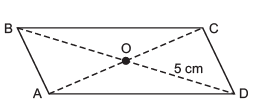Solution: ∵ Diagonals of a parallelogram bisect each other.
∴  OD = OB = 5 cm
or  OB = 5 cm
or  BD = 5 cmx 2 = 10 cm
∵   AC = BD – 2 cm
∴   AC = (10 – 2) cm = 8 cm
or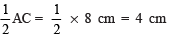or   OA = 4 cm.

Offer running on EduRev: Apply code STAYHOME200 to get INR 200 off on our premium plan EduRev Infinity!

## Mathematics (Maths) Class 8

144 videos|317 docs|48 tests

,

,

,

,

,

,

,

,

,

,

,

,

,

,

,

,

,

,

,

,

,

;